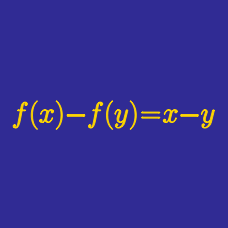Algebra

# Functional Equations - Substitution

Find all functions $$f: \mathbb{R} \backslash \{ 0, 1 \} \rightarrow \mathbb{R}$$ such that

$f \left( \frac{a}{a-1} \right) - 11 f \left( \frac{ a-1} {a} \right) = 0,$

for $$a \neq 0, 1$$.

A function $$f(x)$$ from the positive integers to the reals satisfies
1) $$f(1) = 792$$
2) $$f(1) + f(2) + \ldots + f(n) = n^2 f(n)$$ for any positive integer $$n$$.

What is the value of $$f(11)$$?

Consider the function $$f(x)$$ satisfying

$\left(x^2-x+1\right)f\left(x^2\right)=f(x), f(3)=3.$

If the value of $$f(-9)$$ can be expressed as $$\frac{a}{b},$$ where $$a$$ and $$b$$ are coprime positive integers, what is the value of $$a+7b?$$

A function $$f: \mathbb{R} \backslash \{ 0, 1 \} \rightarrow \mathbb{R}$$ satisfies

$f(x) + f \left( \frac{1}{1-x} \right) = 5 x,$

for $$x \neq 0, 1$$. What is the value of $$40 f( 5)$$?

Suppose that $$f$$ satisfies the following functional equation: $2f(x)+3f\left(\frac{3x+29}{x-3}\right)=100x+80.$ What is the value of $$f(4)?$$

×